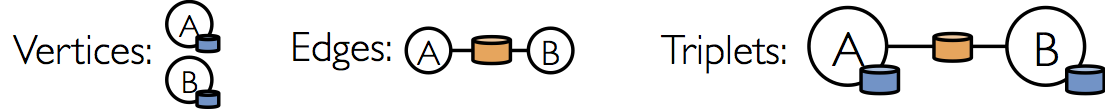# 屬性圖

class VertexProperty()
case class UserProperty(val name: String) extends VertexProperty
case class ProductProperty(val name: String, val price: Double) extends VertexProperty
// The graph might then have the type:
var graph: Graph[VertexProperty, String] = null


class Graph[VD, ED] {
val vertices: VertexRDD[VD]
val edges: EdgeRDD[ED]
}


VertexRDD[VD]EdgeRDD[ED]類別分别繼承和最佳化自RDD[(VertexID, VD)]RDD[Edge[ED]]VertexRDD[VD]EdgeRDD[ED]都支持額外的功能來建立在圖計算和利用内部最佳化。

## 屬性圖的例子val userGraph: Graph[(String, String), String]


// Assume the SparkContext has already been constructed
val sc: SparkContext
// Create an RDD for the vertices
val users: RDD[(VertexId, (String, String))] =
sc.parallelize(Array((3L, ("rxin", "student")), (7L, ("jgonzal", "postdoc")),
(5L, ("franklin", "prof")), (2L, ("istoica", "prof"))))
// Create an RDD for edges
val relationships: RDD[Edge[String]] =
sc.parallelize(Array(Edge(3L, 7L, "collab"),    Edge(5L, 3L, "advisor"),
Edge(2L, 5L, "colleague"), Edge(5L, 7L, "pi")))
// Define a default user in case there are relationship with missing user
val defaultUser = ("John Doe", "Missing")
// Build the initial Graph
val graph = Graph(users, relationships, defaultUser)


val graph: Graph[(String, String), String] // Constructed from above
// Count all users which are postdocs
graph.vertices.filter { case (id, (name, pos)) => pos == "postdoc" }.count
// Count all the edg變s where src > dst
graph.edges.filter(e => e.srcId > e.dstId).count

注意，graph.vertices 返回一個 VertexRDD[(String, String)]，它繼承於 RDD[(VertexID, (String, String))]。所以我們可以用以下scala的case案例類別解構這個元組(tuple)。另一方面，
graph.edges 返回一個包含 Edge[String] 物件的 EdgeRDD。我們也可以使用案例類別建構子，如下例所示。

graph.edges.filter { case Edge(src, dst, prop) => src > dst }.count


SELECT src.id, dst.id, src.attr, e.attr, dst.attr
FROM edges AS e LEFT JOIN vertices AS src, vertices AS dst
ON e.srcId = src.Id AND e.dstId = dst.IdEdgeTriplet 類別繼承於 Edge 類別，並且加入了 srcAttrdstAttr 成员，這兩個成員分別包含來源和目的的屬性。我們可以用以下三元組檢視表產生字串集合用來描述用戶之間的關係。

val graph: Graph[(String, String), String] // Constructed from above
// Use the triplets view to create an RDD of facts.
val facts: RDD[String] =
graph.triplets.map(triplet =>
triplet.srcAttr._1 + " is the " + triplet.attr + " of " + triplet.dstAttr._1)
facts.collect.foreach(println(_)變